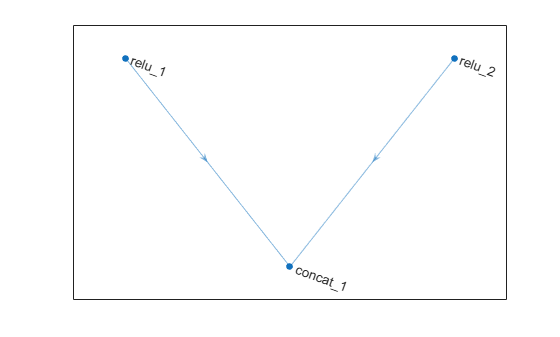# depthConcatenationLayer

Depth concatenation layer

## Description

A depth concatenation layer takes inputs that have the same height and width and concatenates them along the channel dimension.

Specify the number of inputs to the layer when you create it. The inputs have the names `'in1','in2',...,'inN'`, where `N` is the number of inputs. Use the input names when connecting or disconnecting the layer by using `connectLayers` or `disconnectLayers`.

## Creation

### Syntax

``layer = depthConcatenationLayer(numInputs)``
``layer = depthConcatenationLayer(numInputs,'Name',name)``

### Description

example

````layer = depthConcatenationLayer(numInputs)` creates a depth concatenation layer that concatenates `numInputs` inputs along the channel dimension. This function also sets the `NumInputs` property.```

example

````layer = depthConcatenationLayer(numInputs,'Name',name)` also sets the `Name` property.```

## Properties

expand all

Number of inputs to the layer, specified as a positive integer greater than or equal to 2.

The inputs have the names `'in1','in2',...,'inN'`, where `N` is `NumInputs`. For example, if `NumInputs` is 3, then the inputs have the names `'in1','in2'`, and `'in3'`. Use the input names when connecting or disconnecting the layer using the `connectLayers` or `disconnectLayers` functions.

Layer name, specified as a character vector or a string scalar. For `Layer` array input, the `trainNetwork`, `assembleNetwork`, `layerGraph`, and `dlnetwork` functions automatically assign names to layers with the name `''`.

Data Types: `char` | `string`

Input names, specified as `{'in1','in2',...,'inN'}`, where `N` is the number of inputs of the layer.

Data Types: `cell`

Number of outputs of the layer. This layer has a single output only.

Data Types: `double`

Output names of the layer. This layer has a single output only.

Data Types: `cell`

## Examples

collapse all

Create a depth concatenation layer with two inputs and the name `'concat_1'`.

`concat = depthConcatenationLayer(2,'Name','concat_1')`
```concat = DepthConcatenationLayer with properties: Name: 'concat_1' NumInputs: 2 InputNames: {'in1' 'in2'} ```

Create two ReLU layers and connect them to the depth concatenation layer. The depth concatenation layer concatenates the outputs from the ReLU layers.

```relu_1 = reluLayer('Name','relu_1'); relu_2 = reluLayer('Name','relu_2'); lgraph = layerGraph; lgraph = addLayers(lgraph,relu_1); lgraph = addLayers(lgraph,relu_2); lgraph = addLayers(lgraph,concat); lgraph = connectLayers(lgraph,'relu_1','concat_1/in1'); lgraph = connectLayers(lgraph,'relu_2','concat_1/in2'); plot(lgraph)```expand all

## Version History

Introduced in R2017b